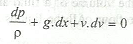# Euler's Equation

The Euler's equation in the differential form for the motion of liquids is given as follows:This equation is based on the following assumptions:

(a) The fluid is non - viscous.

(b) The fluid is homogeneous and in-compressible.

(c) The flow is continuous, steady and along the streamline.

(d) The velocity of flow is uniform over the section.

Note: The Bernoulli's equation is obtained by integrating the above Euler's equation.